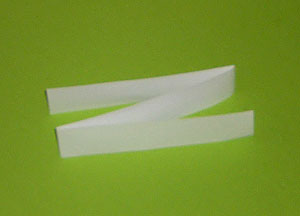Home > Patterns > Activities > Growing fractions

# Growing fractions

You can use patterns to illustrate simple fractions.

Each student will need at least three strips of paper about 2 cm wide and 30 cm long.

Ask students to divide one of their strips into two equal parts. How many folds were required? What is each part called?

Challenge students to divide another strip into three equal parts by folding. Expect a lot of trial and error. Students may come up with the idea of folding a 'Z snake'.The strip folded into three equal parts.

Repeat with folding into four equal parts. Encourage students to fold a 'W snake' rather than re-folding the halves.The strip folded into four equal parts.

Summarise and explain the patterns students observe.

• The parts in the strips are getting smaller.
• The number of parts and the number of folds both increase by one each time.
• The number of folds is always one less than the number of parts.

Ambitious students may continue the pattern to greater numbers of folds.

See Folding Patterns for another patterning activity involving fractions.